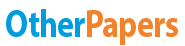# Analysis of Indian Rupee Depreciation Using Swot - Ahp Method

Essay by   •  September 27, 2015  •  Research Paper  •  5,746 Words (23 Pages)  •  1,160 Views

## Essay Preview: Analysis of Indian Rupee Depreciation Using Swot - Ahp Method

Report this essay
Page 1 of 23

Analysis of Indian Rupee Depreciation using SWOT- AHP method

Abstract

The Analytic Hierarchy Process is a popular and widely used method for multi-criteria decision making. One of the main reasons for its popularity is that it allows the usage of qualitative, as well as, quantitative criteria in evaluation. Analytic Hierarchy Process (AHP) approach achieves pairwise comparisons among factors or criteria in order to prioritize them using the eigenvalue calculation. Here the AHP is used to sequence the factors affecting the value of the Indian Rupee, while the factors are determined using SWOT analysis. Strength, Weakness, Opportunities and Threat analysis is used to identify both the internal factors (strength, weakness) and the external factors (opportunities, threat) that affects the exchange rate of the Indian Rupee.

Keywords: Analytic Hierarchy Process, SWOT, exchange rate, Indian Rupee.

Introduction

The value of a currency depends on numerous factors that affect the economy such as imports and exports, inflation, employment, interest rates, growth rate, trade deficit, performance of equity markets, foreign exchange reserves, macroeconomic policies, foreign investment inflows, banking capital, commodity prices and geopolitical conditions. As with other commodities, market forces of demand and supply are the major determinants of the value of rupee against the dollar. In a scenario, when the demand for dollar witnesses an uptrend, the value of rupee in its respect depreciates, which consequently lowers the purchasing power of the rupee.

SWOT analysis is a method that is used to identify all the positive and negative elements of a situation.  SWOT analysis begins with establishing the objective of the project. After establishing the objectives, the various internal and external factors that have a desirable or undesirable effect on achieving the objectives are identified. These factors are categorized into the following four sets

1) Strengths: (internal factors) elements that are considered positive

2) Weaknesses: (internal factors) elements that are considered negative

3) Opportunities: (external factors) elements that are considered positive

4) Threats: (external factors) elements that are considered negative

SWOT analysis is important because they give information on what the positives and negatives will be to achieve an objective.  This analysis facilitates evaluation of various alternatives and thus it makes the decision making process easier.

Analytic Hierarchy Process (AHP) is a structured multiple criteria decision making technique that is used to analyse complex decisions. It was developed by Thomas L. Saaty in the 1970s and has been extensively studied and refined since then. AHP is a structured technique which is used with complex decision-making. The goal is to find out the most valuable decision. While doing so, one looks at a method that would be the most adequate and useful to the users. AHP offers a rational and a sensible method or framework for structuring the problem and quantifying the elements that constitute the problem. The technique used here puts together all these elements and evaluates all the alternatives thereby directing us towards the most efficient solution.

The first step is to construct a hierarchy that would break down the problem statements into different elements. The top most level of the hierarchy is the goal or the problem we are looking to solve. Following this, the second level would have all the criteria that have influence over the objective. Each criterion will have elements that would constitute that particular criterion as sub-criteria.

Once the hierarchy is created, the next step is the relative ranking of all the criteria with respect to one another using the scale shown in Table 1. Similarly, all the sib-criteria under each criterion are also ranked with respect to one another using this same scale. As a result both qualitative and quantitative information can be weighed and compared so as to rank them in order of priority.

 INTENSITY OF IMPORTANCE DEFINITION 1 Equal importance 2 Medium importance 3 Strong importance 4 Very strong importance 5 Absolute importance Reciprocal of the above non-zero numbers If activity i has one of the above non-zero numbers assigned to it when compared with activity j, then jhas the reciprocal value when compared with i

Table 1. Pairwise comparison scale

Let C = {Cj| j = 1, 2 . . . n} be the set of criteria or sub-criteria. Once the pairwise ranking is done, the results can be formed into an (n x n) matrix where every element  [pic 1]is the weight of the criteria i in comparison to j.

A = ()nxn =

Once the matrix A is obtained, it is normalized column-wise and the priority vector (X) is formed which is computed as the average of each rows.

Using the equation,

A.X = .X

is calculated. Also, using the equation below, the value of Consistency Index (CI) is computed.

CI =

The value of the random index (RI) is obtained from the table given below and using this value, the value of Consistency Ratio (CR) is computed.

CR =

If the value of CR is less than or equal to 0.1, it is acceptable. If the value is greater, the procedure has to be repeated again to improve consistency.

 n 1 2 3 4 5 6 7 8 9 10 RI 0 0 0.58 0.9 1.12 1.24 1.32 1.41 1.45 1.49

SWOT analysis generally provides an idea for planning but does not take the relative importance of various elements into consideration. AHP method gives a very structured and quantitative idea to the problem. The SWOT – AHP model combines both these aspects thereby making the whole process of decision making more meaningful. As in the AHP, the first stage of the hierarchy is the objective whereas the second stage here in the SWOT-AHP model are the criteria- Strength, Weakness, Opportunity and Threat. Further, each of these criteria has their own sub-criteria.

...

...